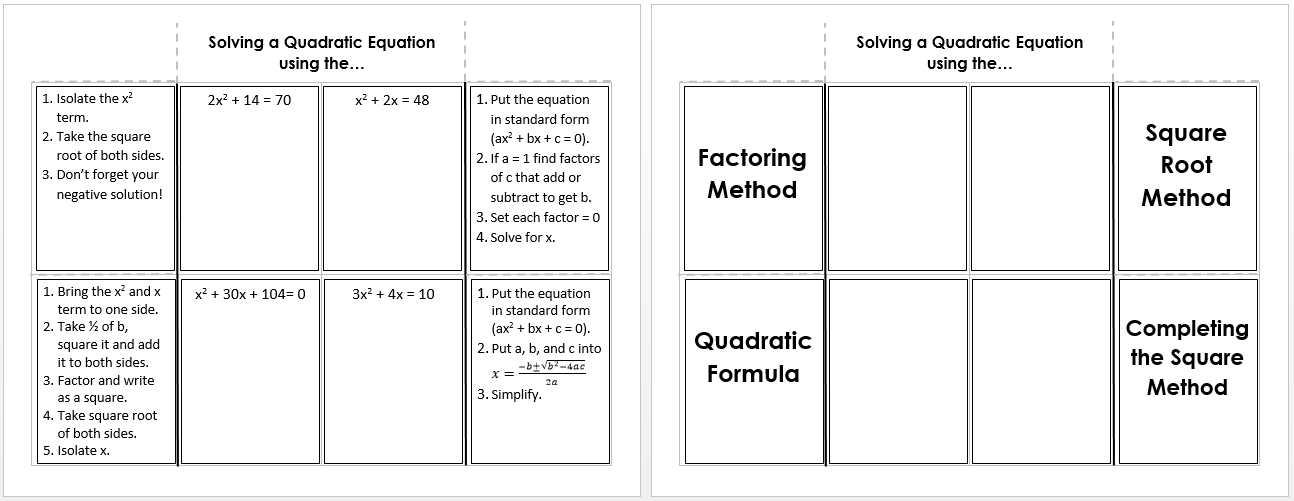# quadratic equations

/Tag:quadratic equations
•Solving Quadratic Equations
Gallery

## Solving Quadratic Equations

There are many ways to solve quadratic equations. The resources below can help you remember key concepts, discover new methods, and fine tune your skills. Solving by factoring and graphing is a worksheet that contains three types of equations - perfect square trinomials, difference of squares, and factoring trinomials to solve. It also has two solving [...]

By | 2017-11-13T22:02:00+00:00 September 25th, 2015|Algebra II, Algebra Tiles, PreCalculus|0 Comments